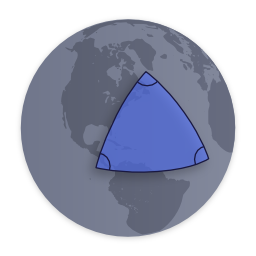# spherical-geometry-js

This library provides classes and functions for the computation of geometric data on the surface of the Earth. Code ported from the Google Maps Javascript API v3

## Usage no npm install needed!

``````<script type="module">
import sphericalGeometryJs from 'https://cdn.skypack.dev/spherical-geometry-js';
</script>``````# Spherical Geometry Library ·This library provides classes and functions for the computation of geometric data on the surface of the Earth.

This library ports a small but useful subset of classes from the Google Maps Javascript API version 3, to use as a separate module or in node. I also try to have readable code, so that you can understand what calculations are being made.

## How to use

``````import * as geometry from 'spherical-geometry-js';
``````

Or import individual modules

``````import { computeArea } from 'spherical-geometry-js';
import computeArea from 'spherical-geometry-js/compute-area';
``````

Notes:

• The API is nearly identical to the Google Maps Javascript API.
• Functions automatically convert coordinate objects into `LatLng`s. See `convertLatLng` for more details.
• All computed lengths are returned in meters.

## API

The full API of the library is described in the typings file.

Classes and libraries ported from the Google Maps Javascript API:

This module tries to maintain full API compatibility with Google Maps so it can be used as a drop-in replacement.

For convenience, `LatLng` includes some extra methods.

``````const latlng = new LatLng(123, 56);
// Alias getters for longitude and latitude

latlng.x === latlng.lng();
latlng.y === latlng.lat();

latlng === latlng.lng();
latlng === latlng.lat();
``````
``````import { equalLatLngs } from 'spherical-geometry-js';

equalLatLngs(latlng1, latlng2) === latlng1.equals(latlng2);
``````

### convertLatLng(like) ⇒ `LatLng`

``````import { LatLng, convertLatLng } from 'spherical-geometry-js';

convertLatLng({ lat: 123, lng: 56 }).equals(new LatLng(123, 56));
convertLatLng([56, 123]).equals(new LatLng(123, 56));
convertLatLng({ x: 56, y: 123 }).equals(new LatLng(123, 56));
``````

Helper function that tries to convert and object into a `LatLng`. Tries a few different methods:

1. If `instanceof LatLng`, clone the object and return it.

2. If it has `lat` and `lng` properties...

2a. if the properties are functions (like Google `LatLng`s), use the `lat()` and `lng()` values as latitude and longitude.

2b. otherwise get `lat` and `lng`, parse them as floats and use them.

3. If it has `lat` and `long` properties, parse them as floats use them.

4. If it has `lat` and `lon` properties, parse them as floats use them.

5. If it has `latitude` and `longitude` properties, parse them as floats use them.

6. If it has number values for `0` and `1` (aka an array of two numbers), use `1` as latitude and `0` as longitude.

7. If it has `x` and `y` properties, try using `y` as latitude and `x` and longitude.